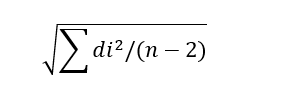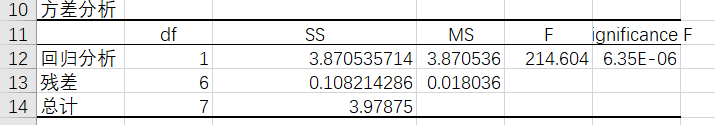3256字，阅读需时11分钟

【摘要：课程通过刀具磨损回归分析案例，借助于EXECL分析工具，讲述数据分析的结果表达——数据图表的使用，重点讲述了回归分析结果的散点图、趋势线和回归统计表，并编写Python程序绘制散点图和趋势线。】（1）选择要分析的数据，如下图所示：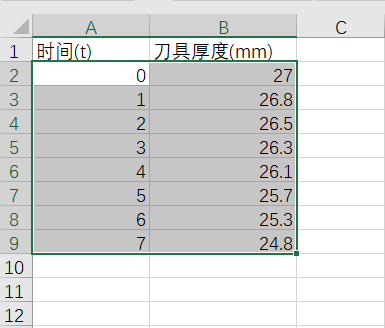（2）展开【数据】标签栏，在【数据】标签栏中选择“数据分析”功能，如下图所示：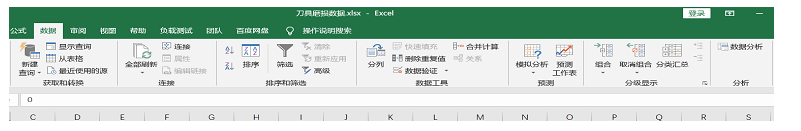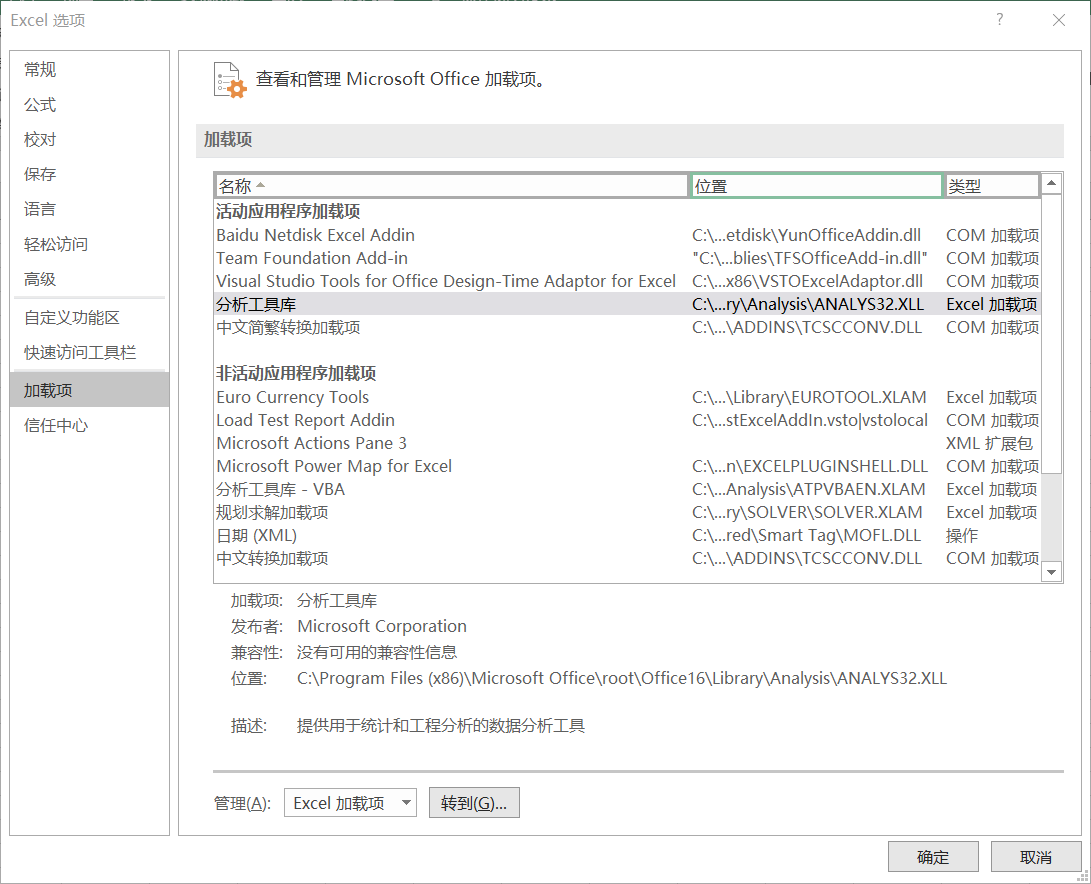（3）在数据分析对话框，选择回归分析，如下图所示：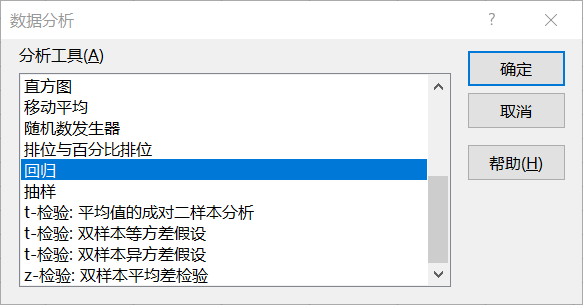（4）在回归分析对话框，填入需要进行回归分析的数据区域和输出选项。X值输入区域为时间数据，Y值输入区域为刀具厚度数据。输出的图表选择残差、线性拟合图和标准残差。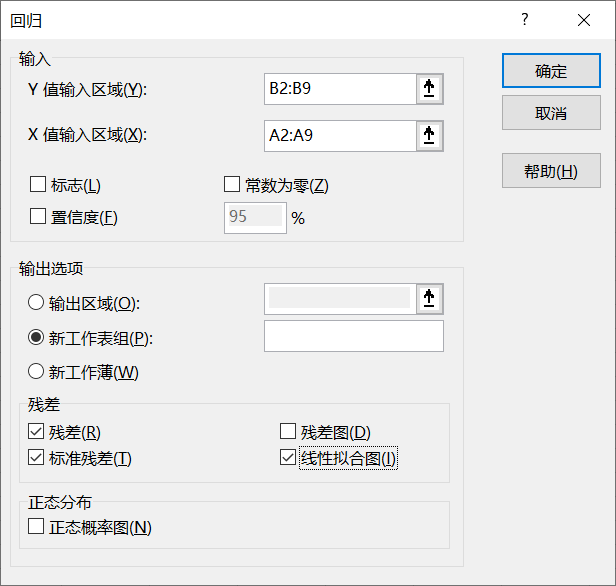（5）回归分析结果的数据图表会出现在新工作表组：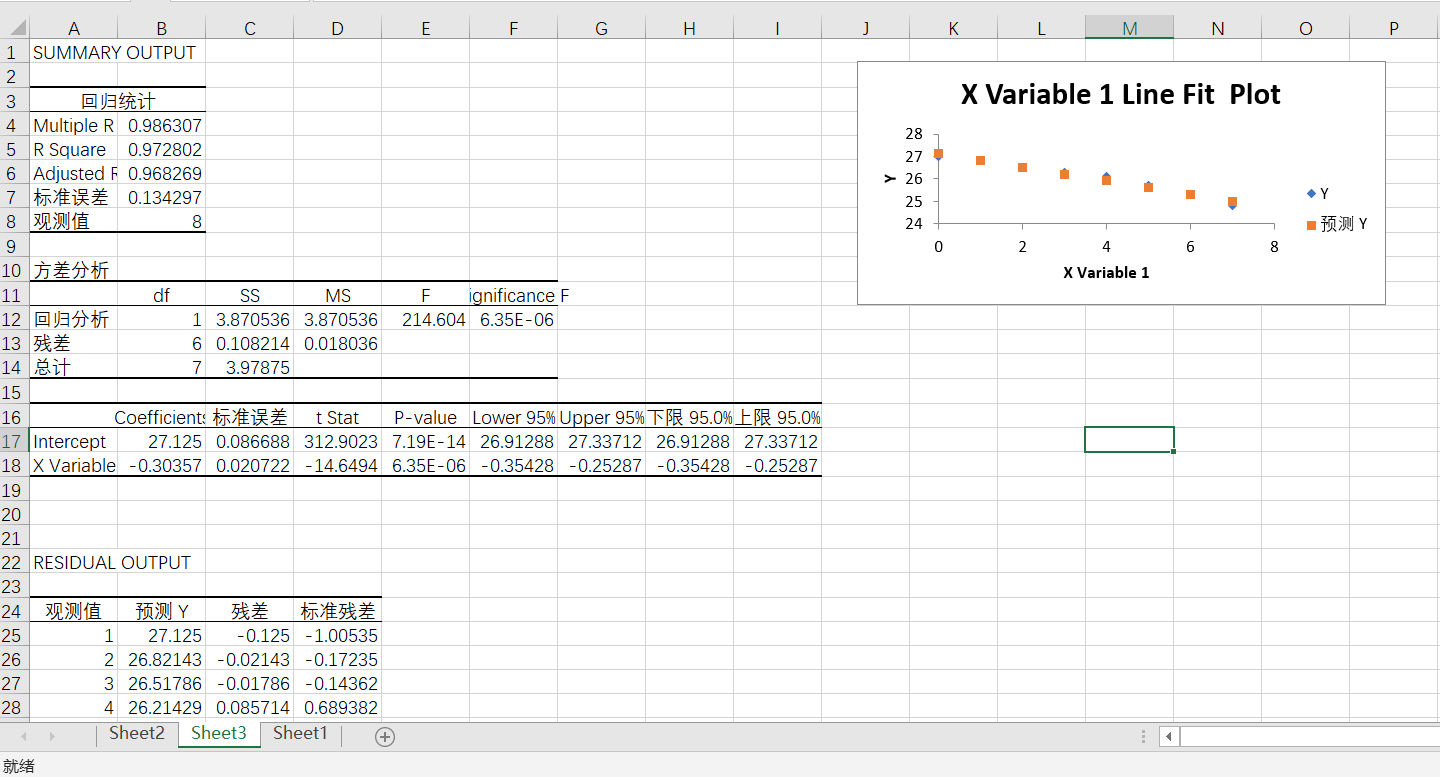（6）观察EXECL给出的分析图表，并没有给出与数据关系近似的线性函数。选择散点图，单击散点图右侧的“+”按钮，添加趋势线，并设置趋势线格式，在“设置趋势线格式”窗口，选择“选中公式”选项。趋势线和趋势线函数会显示在散点图内。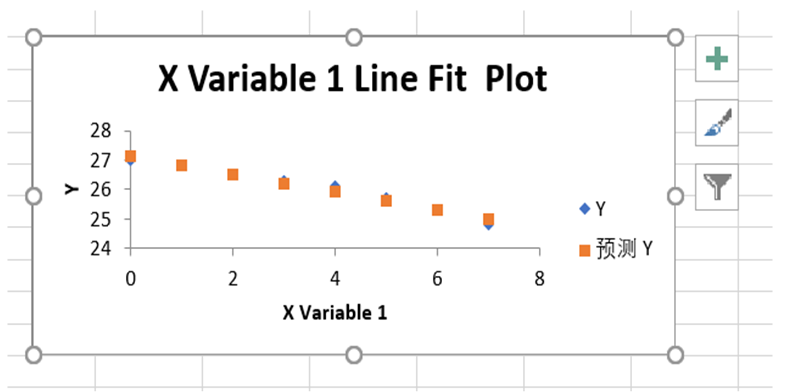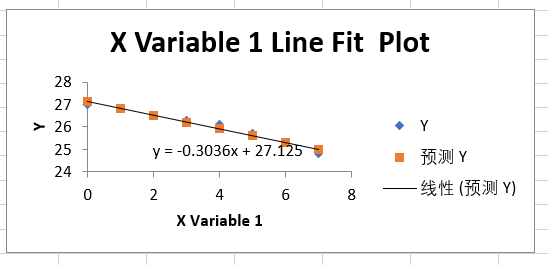（案例代码见unit1\ case05.py）

```# 导入matplotlib库
import numpy as np
# 导入matplotlib库
import matplotlib.pyplot as plt

# 定义绘制趋势线函数
# x,y为实验数据，n表示n次函数
def plot_trendline(x,y,n):
# 计算实验数据的拟合函数系数
z = np.polyfit(x,y,n)
# z为拟合函数系数向量，返回拟合函数
p = np.poly1d(z)
# 绘制拟合函数图形
# r--为红色点划线
plt.plot(x,p(x),"r--")

# 定义实验数据
x = [0,1,2,3,4,5,6,7]
y = [27,26.8,26.5,26.3,26.1,25.7,25.3,24.8]
# 设置散点图标量和颜色
plt.scatter(x,y, s=40, c='green')

# 设置散点图标题
plt.title("X Variable 1 Line Fit  Plot", fontsize=20)
# 设置散点图x轴标题
plt.xlabel("X Variable 1", fontsize=16)
# 设置散点图y轴标题
plt.ylabel("Y", fontsize=16)
plot_trendline(x,y,1)
#显示绘制的图形
plt.show()```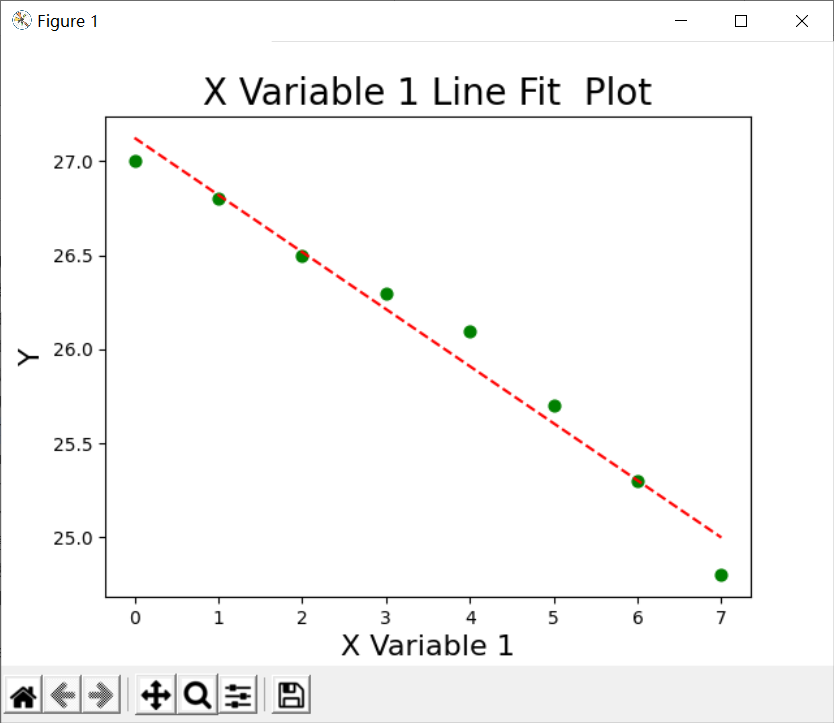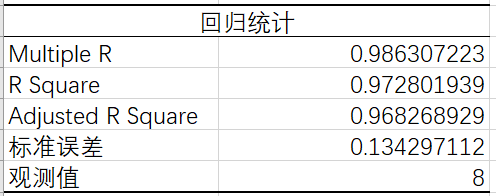Multiple R是刀具厚度（y）和时间（x）的相关系数，该值一般在-1~1之间，该值为正数时为正相关，为负数时为负相关，为0时没有相关性。该值越接近于1，说明x和y正相关越强，该值越接近于-1，说明负相关越强。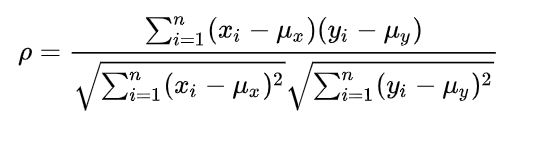（案例代码见unit1\ case04.py）

```# 导入pandas库
import pandas as pd

# 定义DataFrame数据列
df = pd.DataFrame({'time':[0,1,2,3,4,5,6,7],
'thick':[27,26.8,26.5,26.3,26.1,25.7,25.3,24.8]})

# 输出DataFrame数据列
print(df)
# 计算DataFrame数据列的相关系数
print(df.corr())```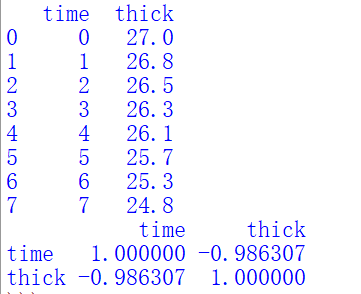R Square为线性函数的确定系数，用于衡量线性回归模型（近似的线性函数），预测因变量随自变量变化的可靠程度。该值一般在0~1之间，该值越接近于1，说明回归分析给出的回归模型更可靠。R Square的值为相关系数的平方。

1-(n-1)(1-r)/(n-p-1)